# Graphical Method to Find the Solution Set of a Quadractic Equation

1. ေပးထားေသာ Function ကို y ဟုထားပါ။
2. y=0 ထားၿပီး X-axis ျဖတ္မွတ္မ်ားကို ရွာပါ။
3. x=0 ဟုထားၿပီး Y-axis ျဖတ္မွတ္ကို ရွာပါ။
4. ျဖတ္မွတ္မ်ားကို အသံုးျပဳၿပီး smooth parabola ဆြဲပါ။
5. ေပးေထားေသာ inequation sign ကို ၾကည့္ၿပီး solution set ကို ဆံုးျဖတ္ပါ။

Example 1
Use a graphical method, to find the solution set of the inequation 12 - 5x - 2x2 ≥ 0 and illustrate it on the number line.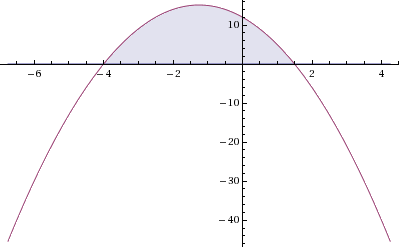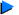Solution
Let y=12 - 5x - 2x2
When y = 0,
12 - 5x - 2x2 = 0
(4 + x)(3 - 2x) = 0
x = -4 or x = 3/2
Therefore, the graph cuts the X-axis at (-4,0) and (3/2,0).
When x = 0, y = 12
Therefore, the graph cuts the Y-axis at (0,12).

The solution set of
12 - 5x - 2x2 ≥ 0 is {x/-4 ≤ x ≤ 3/2}.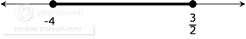Example 2

Find the solution set of the inequation 3x2 < x2 - x + 3 by graphical method and illustrate it on the number line.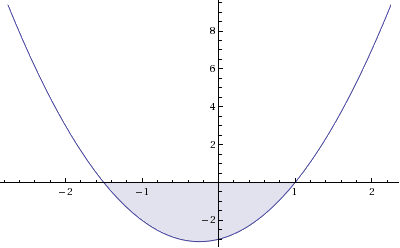Solution
3x2 < x2 - x + 3
2x2 + x - 3 <0
Let y=
2x2 + x - 3
When y = 0,
2x2 + x - 3 = 0
(
2x + 3)(x - 1) = 0
x = -
3/2 or x = 1
Therefore, the graph cuts the X-axis at (-
3/2,0) and (1,0).
When x = 0, y = -3
Therefore, the graph cuts the Y-axis at (0,-3).

The solution set of
3x2 < x2 - x + 3 is {x/-3/2 < x <1}.>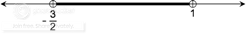Example 3
Find the solution set of the inequation 12x2 10 - 7x by graphical method and illustrate it on the number line.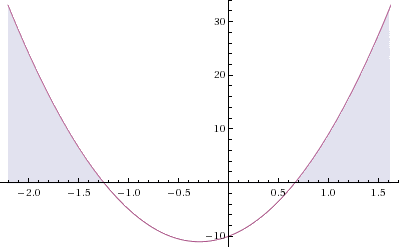Solution
12x2 10 - 7x
12x2 + 7x - 10 0
Let y=
12x2 + 7x - 10
When y = 0,
12x2 + 7x - 10= 0
(
4x + 5)(3x - 2) = 0
x = -
5/4 or x = 2/3
Therefore, the graph cuts the X-axis at
(- 5/4,0) and (2/3,0).
When x = 0, y = -10
Therefore, the graph cuts the Y-axis at (0,-10).

The solution set of
3x2 < x2 - x + 3 is {x/ x
- 5/4 or x 2/3}.